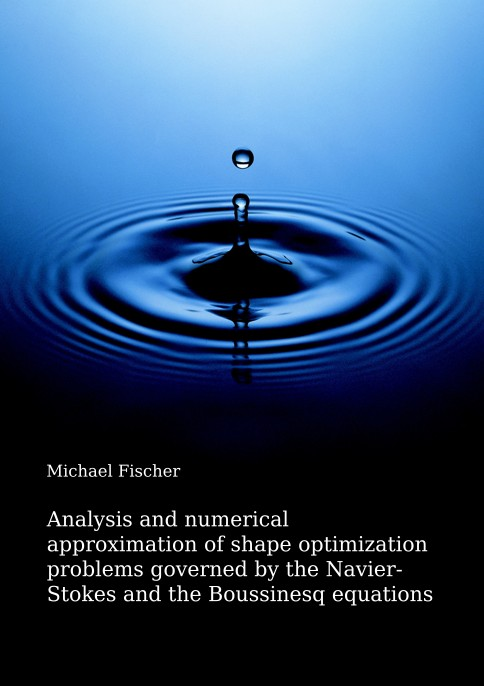Datenbestand vom 30. September 2022aktualisiert am 30. September 2022

# ISBN 978384393268484,00 € inkl. MwSt, zzgl. Versand

978-3-8439-3268-4, Reihe Mathematik

Michael Fischer
Analysis and numerical approximation of shape optimization problems governed by the Navier-Stokes and the Boussinesq equations

249 Seiten, Dissertation Technische Universität Darmstadt (2017), Softcover, B5

## Zusammenfassung / Abstract

Shape optimization problems in connection with complex fluids play a major role not only in theoretical and practical mathematics but also in physics and engineering sciences. They belong to the class of shape optimization problems with partial differential equations and they are an active field of research in mathematical science. Pioneering results concerning the mathematical investigation of shape optimization problems in connection with fluid dynamics have been proposed by Pironneau, Simon and Bello et al. In this thesis we consider shape optimization problems governed by the Navier-Stokes and the Boussinesq equations, which describe the time dependent flow of particles and their temperature distribution.

Due to the huge amount of optimization variables in shape optimization problems with partial differential equations, modern gradient based optimization methods make use of the well-known adjoint approach for optimal control problems and require the differentiability of the control-to-state operator associated with the differential equation in a sufficiently strong topology. Therefore, the question of differentiability of this operator resulting from an application of the method of mapping by Murat and Simon to the incompressible instationary Boussinesq equations is addressed in this thesis. Furthermore, for a rigorous application of the adjoint approach we investigate the analytic properties of the adjoint linearized Boussinesq operator. Those theoretical results are also validated by implementing an adjoint based optimization framework in order to practically solve a shape optimization problem associated with the Boussinesq equations.

In this thesis we furthermore apply the method of mapping to the discrete system resulting from an application of a P1/P1 discretization to the Navier-Stokes equations and investigate the differentiability properties of the corresponding discrete control-to-state operator. Here, the main focus is put on the independence of the spatial and time discretization sizes in the differentiability results, such that the results remain stable for decreasing discretization sizes and a convergence process through the infinite dimensional solution can be considered.